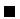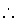Next: Negation as Failure Up: The Prolog Language Previous: Meta-Logical Predicates

Subsections

# Meta-calls (Higher Order)

Meta-calls allow performing on the fly'' calls to terms which, if they correspond literally to the name of a program predicate, will be executed as program code. The basic meta-call predicate is call/1, which accepts a term and calls it as if it appeared in the program text. Thus, call(p(X)) is equivalent to the appearance of p(X) in the program text. The argument X of call(X) (and this is really where the power of meta-calls is) does not need to be explicitly present in the source code, but only correctly instantiated at run-time.

Example 4.5   The following code implements a naïve apply/2 which takes as argument an atom (which should be a predicate name) and a list of terms (which are intended to be the arguments of the predicate) and makes the corresponding call (i.e., apply(foo, [1, X, best])) makes the call foo(1, X, best):

apply(Atom, ListArgs):-
Term =.. [Atom|ListArgs],
call(Term).


(Incidentally, this is one of the few cases where the use of =.. / 2 is justified). This code allows constructing calls to predicates which are not known at compile time.The more important use of meta-calls is to implement general predicates which perform tasks using the result of calls as data. The best known of them are the all-solutions predicates and the negation.All solutions'' predicates gather in a list all the solutions to a query. The more relevant are findall/3, bagof/3, and setof/3.

#### findall(Term, Goal, List)

leaves in List an instance of Term for each success of Goal. We will use the following program as example:

p(a, a).
p(b, a).
p(1, 1).
p(2, b).
p(3, 1).


The following queries collect all solutions for calls to p/2. Note that duplicates appear in the solutions list:

?- findall(A, p(A, B), L).
L = [a,b,1,2,3] ?

yes
?- findall(B, p(A, B), L).
L = [a,a,1,b,1] ?

yes
?- findall(A, p(A, 1), L).
L = [1,3] ?

yes
?- findall(example(A, B), p(A, B), L).
L = [example(a,a),example(b,a),example(1,1),example(2,b),example(3,1)] ?

yes


findall/3 will return the empty list if no solutions are found for the goal. It ignores all variables in the query which do not appear in the term whose instances are collected (i.e., solutions are gathered for all bindings of these variables). The predicate bagof/3 allows (selective) backtracking on the free variables of the goal:

?- bagof(A, p(A, B), L).
B = 1, L = [1,3] ? ;
B = a, L = [a,b] ? ;
B = b, L =  ? ;

no
?- bagof(A, B^p(A, B), L).
L = [a,b,1,2,3] ?


In the second case we are explicitly signaling that we do not want to take into account backtracking for different bindings of B. Also, bagof/3 will fail (instead of reporting an empty list) if the called predicate fails.

Last, the meta-predicate setof/3 behaves as bagof/3, but in addition the returned list is sorted and has no repetitions.

Note that no bindings are returned for the variables appearing in the first argument of all three predicates.Next: Negation as Failure Up: The Prolog Language Previous: Meta-Logical Predicates
MCL
1998-12-03